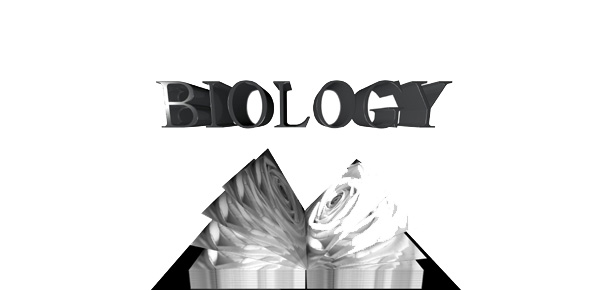# Biology Cp - Unit 2

26 Questions | Total Attempts: 120SettingsPrentice Hall: Biology The Living World

• 1.
Negatively charged sub-component of atom:
• A.

Electron

• B.

Proton

• C.

Neutron

• 2.
An element is matter that contains only one type of:
• A.

Ion

• B.

Atom

• C.

Isotope

• 3.
The atomic number is equal to the number of:
• A.

Neutrons

• B.

Protease

• C.

Protons

• 4.
The mass number is equal to:
• A.

The number of protons plus the number of neutrons

• B.

The number of the element minus neutrons

• C.

The ionic number increased by 2

• 5.
Atoms with the same Atomic Number but different numbers of neutrons are called:
• A.

Zwitterions

• B.

Isotopes

• C.

Anions

• 6.
An atom with an unequal number of protons and electrons is called a(n):
• A.

Ion

• B.

Isotope

• C.

Deuterium

• 7.
A positive charged ion is called a(n):
• A.

Zwitterion

• B.

Anion

• C.

Cation

• 8.
A negatively charged ion is called a(n):
• A.

Anion

• B.

Cation

• C.

Zwitterion

• 9.
This particle is a combination of two or more atoms:
• A.

Noble gas

• B.

Isotope

• C.

Element

• 10.
These substances are to the far right of the Period Chart of Elements, and represent elements that have the most stable electron configurations, with full valence electron shells:
• A.

Organic Salts

• B.

Noble Gasses

• C.

Electron Clouds

• 11.
The carbons in Butane (Butane has 4 Carbon atoms and 10 Hydrogen atoms) are held together by this type of bond:
• A.

Transient

• B.

Ionic

• C.

Covalent

• 12.
This type of ion has a positive charge at one end and a negative charge at the other, giving it unique chemical properties and reactivity:
• A.

Zwitterion

• B.

Isotope

• C.

Deuterium

• 13.
This category of electron shell has one orbital that can hold two electrons:
• A.

S

• B.

P

• C.

D

• 14.
A mixture is when two or more distinct substances are mixed together but do not chemically combined.  Which of the following statements is also true of mixtures?  (Choose the best answer.)
• A.

A solution is a homogenous mixture in which a solute has completely dissolved in the solvent

• B.

A homogenous mixture is one with substances evenly distributed & A heterogenous mixture is one with substances unevenly distributed

• C.

Both A & B are true

• 15.
A suspension is a heterogeneous mixture in which the suspended particles are too large to be evenly distributed, and will eventually settle out due to gravity, unless continually stirred or agitated.
• A.

True

• B.

False

• 16.
Acids tend to pull electrons off other substances, while bases tend donate electrons.
• A.

True

• B.

False

• 17.
HCl (Hydrochloric Acid) is an ionic combination of Hydrogen and Chlorine, and is a POWERFUL BASE that helps digest food in the stomach.
• A.

True

• B.

False

• 18.
A pH of 7 is neutral.
• A.

True

• B.

False

• 19.
These molecules store large amounts of energy in the body:
• A.

Lipids (fats)

• B.

Sugars (including glucose)

• C.

Amino Acids

• 20.
These molecules are the body's main source of energy.
• A.

Fiber

• B.

Carbohydrates

• C.

Proteins

• 21.
Carbon tends to form 3 covalent bonds with other organic compounds.HINT:  Carbon has an atomic number of 6, while the nearest noble gas is neon with an atomic number of 10.
• A.

True

• B.

False

• 22.
Energy and mass are both destroyed during chemical reactions.
• A.

True

• B.

False

• 23.
A black hole is a poorly understood phenomenon that may violate the natural laws of Conservation of Mass and Conservation of Energy.
• A.

True

• B.

False

• 24.
Proteins are made up of specific combinations of Amino Acids.
• A.

True

• B.

False

• 25.
Kinetic energy is the energy of motion.  Potential energy is energy of relative position.
• A.

True

• B.

False

Related TopicsBack to top